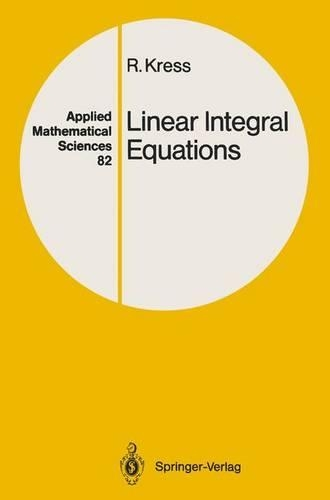•Linear Integral Equations - Applied Mathematical Sciences 82 (Paperback)

(author)
£72.00
Paperback Published: 07/01/2012
• Not available

This product is currently unavailable.

Up till now there has been no text on integral equations adequately combining theory, applications and numerical methods: this book sets out to cover each of these areas with the same weighting. The first part provides the basic Riesz-Fredholm theory for equations of the second kind with compact operators in dual systems, including all functional analytic concepts necessary. The second part then illustrates the classical applications of integral equation methods to boundary value problems for the Laplace equation and the heat equation as one of the main historical sources for the development of integral equations, and also introduces Cauchy type singular integral equations. The third part describes the fundamental ideas for the numerical solutions of integral equations. In the final part, ill-posed integral equations of the first kind and their regularization are studied in a Hilbert space setting. So that the book is accessible not only to mathematicians but also to physicists and egineers, it is kept as self-contained as possible, requiring only a solid foundation in differential and integral calculus and, for some parts in complex function theory. Some background in functional analysis will be helpful, but the basic concepts needed are reviewed or developed within the book: the reader must of course be willing to accept the modern functional analytic language for describing the theory and the numerical solution of integral equations.

Publisher: Springer-Verlag Berlin and Heidelberg GmbH & Co. KG
ISBN: 9783642971488
Weight: 480 g
Dimensions: 234 x 156 x 16 mm
Edition: Softcover reprint of the original 1st ed. 198